# Material Dispersion

In these two examples, we will perform simulations with a frequency-dependent dielectric ε(ω), corresponding to material dispersion. See Materials for more information on how material dispersion is supported.

### Reflectance Spectrum of Air-Silica Interface

We will compute the broadband reflectance spectrum at normal incidence for a planar interface of vacuum/air and fused quartz. As a validation, we will compare the simulated result with the analytic reflectance computed using the Fresnel equations. This is similar to the example in Tutorial/Basics/Angular Reflectance Spectrum of a Planar Interface which involved computing the angular reflectance spectrum at a single wavelength.

The wavelength-dependent, lossless permittivity of fused quartz, measured experimentally at 20°C, can be approximated by the Sellmeier equation:

The wavelength λ is in units of microns. This equation is valid from 0.21 to 6.7 μm. The Sellmeier form for the permittivity of fused quartz can be imported into Meep as a Lorentzian susceptibility via a slight reorganization to convert the wavelength dependence into frequency as described in Materials. This is implemented in the materials library.

The simulation involves a 1d cell. A planewave current source with a pulsed profile spanning visible wavelengths of 0.4 to 0.8 μm is normally incident on the quartz from air. The reflectance is computed using the convention of two separate runs: (1) an empty cell to obtain the incident power, and (2) with the quartz to obtain the reflected power. The details of this type of calculation are described in Tutorial/Basics/Transmittance Spectrum of a Waveguide Bend. The grid resolution, and by direct extension the time resolution via the Courant condition, must be made sufficiently fine to obtain agreement with the analytic results and to ensure numerical stability. Coarse resolutions may lead to field instabilities.

The simulation script is in examples/refl-quartz.ctl.

(set-param! resolution 200) ; pixels/μm

(define-param sz 10)
(set! geometry-lattice (make lattice (size no-size no-size sz)))
(set! dimensions 1)

(define lambda-min 0.4)
(define lambda-max 0.8)
(define fmax (/ lambda-min))
(define fmin (/ lambda-max))
(define fcen (* 0.5 (+ fmax fmin)))
(define df (- fmax fmin))

(define dpml 1.0)
(set! pml-layers (list (make pml (thickness dpml))))

(set! k-point (vector3 0 0 0))

(set! sources (list (make source (src (make gaussian-src (frequency fcen) (fwidth df))) (component Ex) (center 0 0 (+ (* -0.5 sz) dpml)))))

(define-param empty? true)

(if (not empty?)
(set! geometry (list (make block (size infinity infinity (* 0.5 sz)) (center 0 0 (* 0.25 sz)) (material fused-quartz)))))

(define nfreq 50)
(define refl (add-flux fcen df nfreq (make flux-region (center 0 0 (* -0.25 sz)))))

(if (not empty?) (load-minus-flux "refl-flux" refl))

(run-sources+ (stop-when-fields-decayed 50 Ex (vector3 0 0 (+ (* -0.5 sz) dpml)) 1e-9))

(if empty? (save-flux "refl-flux" refl))

(display-fluxes refl)


The following Bash shell script runs the two simulations, pipes the output to a file, and extracts the flux data into a separate file.

#!/bin/bash

meep empty?=true refl-quartz.ctl |tee flux0.out
grep flux1: flux0.out |cut -d , -f2- > flux0.dat

meep empty?=false refl-quartz.ctl |tee flux.out
grep flux1: flux.out |cut -d , -f2- > flux.dat


A plot of the reflectance spectrum based on the simulated data and the analytic Fresnel equations is generated using the Octave/Matlab script below. The plot is shown in the accompanying figure. There is agreement between the simulated and analytic results. Note that the reflectance spectra is plotted as a function of wavelength, not frequency from which the Meep data is obtained. Thus, the data points are not equally spaced: the spacing is smaller at low wavelengths (high frequencies) than at high wavelengths (low frequencies).

f0 = dlmread("flux0.dat",",");

wvls = 1./f(:,1);
R_meep = -f(:,2)./f(:,2);

eps_quartz = @(l) 1+(0.6961663*l.^2)./(l.^2-0.0684043^2)+(0.4079426*l.^2)./(l.^2-0.1162414^2)+(0.8974794*l.^2)./(l.^2-9.896161^2);
R_fresnel = @(l) abs((1-eps_quartz(l).^0.5)./(1+eps_quartz(l).^0.5)).^2;

plot(wvls,R_meep,'bo-',wvls,R_fresnel(wvls),'rs-');
xlabel("wavelength (μm)");
ylabel("reflectance");
legend("meep","analytic");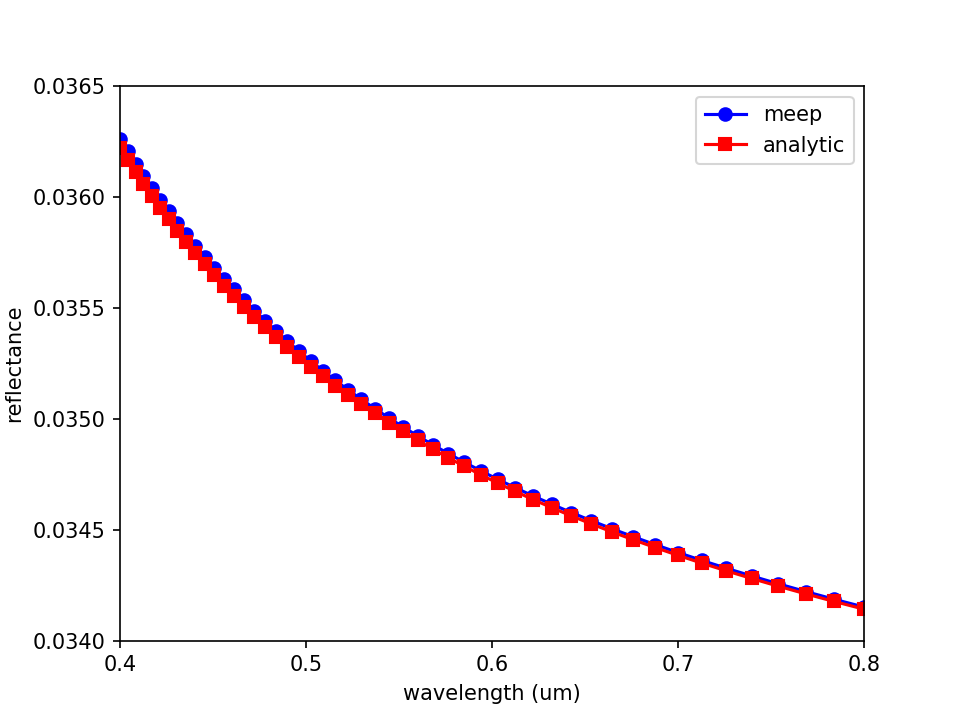### Permittivity Function of an Artificial Dispersive Material

We will model a uniform medium of an artificial dispersive material. From the dispersion relation ω(k), we will compute the numerical ε(ω) via the formula:

We will then compare this with the analytical ε(ω) that we specified. The simulation script is in examples/material-dispersion.ctl.

Since this is a uniform medium, our computational cell can actually be of zero size (i.e. one pixel), where we will use Bloch-periodic boundary conditions to specify the wavevector k.

(set! geometry-lattice (make lattice (size no-size no-size no-size)))
(set-param! resolution 20)


We will then fill all space with a dispersive material:

(set! default-material
(make dielectric (epsilon 2.25)
(E-susceptibilities
(make lorentzian-susceptibility
(frequency 1.1) (gamma 1e-5) (sigma 0.5))
(make lorentzian-susceptibility
(frequency 0.5) (gamma 0.1) (sigma 2e-5))
)))


corresponding to the dielectric function:

The real and imaginary parts of this dielectric function ε(ω) are plotted below: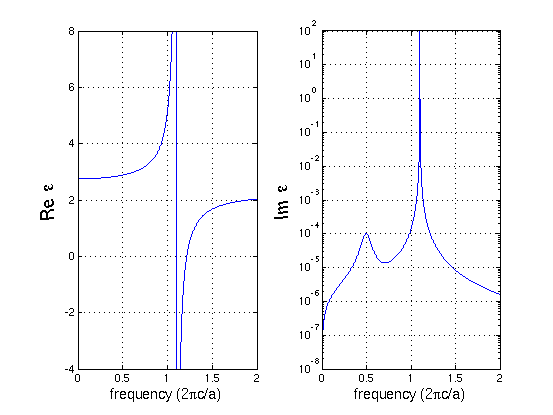We can see that the f=1.1 resonance causes a large change in both the real and imaginary parts of ε around that frequency. In fact, there is a range of frequencies from 1.1 to 1.2161 where ε is negative. In this range, no propagating modes exist — it is actually a kind of electromagnetic band gap associated with polariton resonances in a material. For more information on the physics of such materials, see e.g. Chapter 14 of Introduction to Solid State Physics by C. Kittel.

On the other hand, the f=0.5 resonance, because the sigma numerator is so small, causes very little change in the real part of ε. Nevertheless, it generates a clear peak in the imaginary part of ε, corresponding to a resonant absorption peak.

Now, we'll set up the rest of the simulation. We'll specify a broadband $E_z$-polarized Gaussian source, create a list of k wavevectors that we want to compute ω(k) over, and compute the associated frequencies by using the run-k-points function:

(define-param fcen 1.0)
(define-param df 2.0)
(set! sources (list (make source
(src (make gaussian-src (frequency fcen) (fwidth df)))
(component Ez) (center 0 0 0))))
(define-param kmin 0.3)
(define-param kmax 2.2)
(define-param k-interp 99)
(define kpts (interpolate k-interp (list (vector3 kmin) (vector3 kmax))))
(define all-freqs (run-k-points 200 kpts)) ; a list of lists of frequencies


The run-k-points function returns a list of lists of frequencies — one list of complex frequencies for each k point — which we store in the all-freqs variable. Finally, we want to loop over this list and print out the corresponding ε via the ratio (ck/ω)$^2$ as described above. To do this, we will use the Scheme map function, which applies a given function to every element of a list (or lists), and since we have a list of lists we'll actually nest two map functions:

(map (lambda (kx fs)
(map (lambda (f)
(print "eps:, " (real-part f) ", " (imag-part f)
", " (sqr (/ kx f)) "\n"))
fs))
(map vector3-x kpts) all-freqs)


Alternatively we could just read all of the frequencies into Octave/Matlab and compute the ratios there. After running the program with

unix% meep material-dispersion.ctl | tee material-dispersion.out


we can then grep for the frequencies and the computed dielectric function, and plot it. First, let's plot the dispersion relation ω(k) for the real part of ω: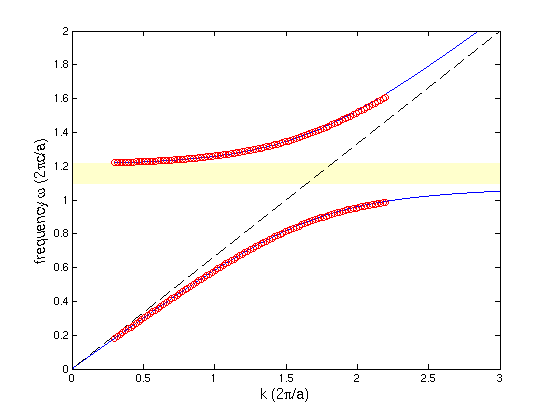The red circles are the computed points from Meep, whereas the blue line is the analytical band diagram from the specified ε(ω). As you can see, we get two bands at each k, separated by a polaritonic gap (shaded yellow). This dispersion relation can be thought of as the interaction (anti-crossing) between the light line of the ambient ε=2.25 material (dashed black line) and the horizontal line corresponding to the phonon resonance.

Similarly, the computed and analytical real parts of the dielectric function are given by: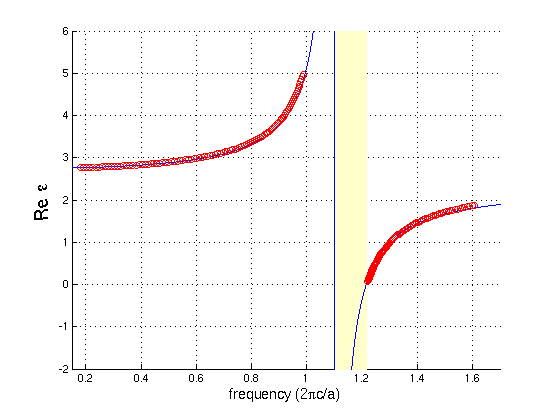which shows excellent agreement between the analytical (blue line) and numerical (red circles) calculations. The imaginary part, however, is more subtle: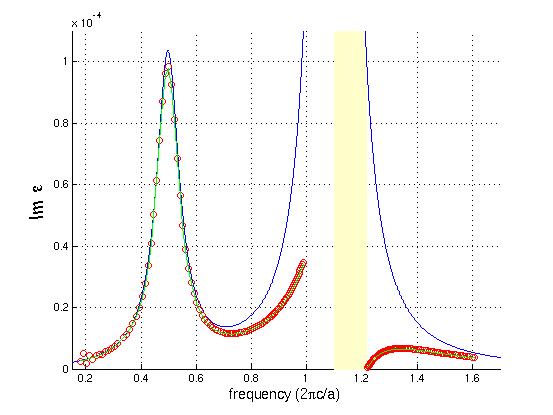The blue line is the analytical calculation from above and the red circles are the numerical value from Meep — why is the agreement so poor? There is nothing wrong with Meep, and this is not a numerical error. The problem is simply that we are comparing apples and oranges.

The blue line is the analytical calculation of ε(ω) for a real frequency ω which corresponds to solutions with a complex wavevector k, whereas Meep is computing ε at a complex ω for a real wavevector k. So, the correct comparison is to plug Meep's complex ω into the analytical formula for ε(ω), which results in the green lines on the graph that fall almost on top of the red circles.

Why did our comparison of the real part of ε look so good, then? The reason is that ε(ω) at real and complex values of ω are closely related by the analytic properties of ε. In particular, because ε is an analytic function on the real-ω axis, adding a small imaginary part to ω as we are doing here does not change ε by much. The losses are small for all of the computed k points. The change was only significant for the imaginary ε because the imaginary ε was small to begin with.